# AKT-09/HW2

Solve the following problems and submit them in class by November 3, 2009:

Problem 1. Let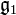${\mathfrak g}_1$ and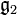${\mathfrak g}_2$ be finite dimensional metrized Lie algebras, let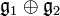${\mathfrak g}_1\oplus{\mathfrak g}_2$ denote their direct sum with the obvious "orthogonal" bracket and metric, and let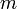$m$ be the canonical isomorphism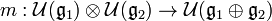$m:{\mathcal U}({\mathfrak g}_1)\otimes{\mathcal U}({\mathfrak g}_2)\to{\mathcal U}({\mathfrak g}_1\oplus{\mathfrak g}_2)$. Prove that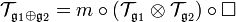${\mathcal T}_{{\mathfrak g}_1\oplus{\mathfrak g}_2} = m\circ({\mathcal T}_{{\mathfrak g}_1}\otimes{\mathcal T}_{{\mathfrak g}_2})\circ\Box$,

where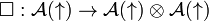$\Box:{\mathcal A}(\uparrow)\to{\mathcal A}(\uparrow)\otimes{\mathcal A}(\uparrow)$ is the co-product and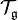${\mathcal T}_{{\mathfrak g}}$ denotes the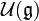${\mathcal U}({\mathfrak g})$-valued "tensor map" on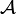${\mathcal A}$. Can you relate this with the first problem of HW1?

Problem 2.

1. Find a concise algorithm to compute the weight system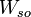$W_{so}$ associated with the Lie algebra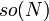$so(N)$ in its defining representation.
2. Verify that your algorithm indeed satisfies the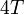$4T$ relation.

Problem 3. The Kauffman polynomial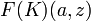$F(K)(a,z)$ (see [Kauffman]) of a knot or link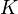$K$ is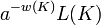$a^{-w(K)}L(K)$ where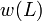$w(L)$ is the writhe of$K$ and where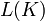$L(K)$ is the regular isotopy invariant defined by the skein relations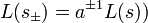$L(s_\pm)=a^{\pm 1}L(s))$

(here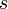$s$ is a strand and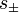$s_\pm$ is the same strand with a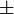$\pm$ kink added) and

Failed to parse (unknown function\backoverslash): L(\backoverslash)+L(\slashoverback) = z\left(L(\smoothing)+L(\hsmoothing)\right)

and by the initial condition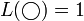$L(\bigcirc)=1$. State and prove the relationship between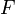$F$ and$W_{so}$.

Mandatory but unenforced. Find yourself in the class photo and identify yourself as explained in the photo page.

[Kauffman] ^  L. H. Kauffman, An invariant of regular isotopy, Trans. Amer. Math. Soc. 312 (1990) 417-471.